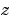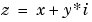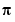MATLAB Function Referencelog

Natural logarithm

Syntax

• ```Y =` `log(X)
```

Description

The `log` function operates element-wise on arrays. Its domain includes complex and negative numbers, which may lead to unexpected results if used unintentionally.

```Y = log(X) ``` returns the natural logarithm of the elements of `X`. For complex or negative, where, the complex logarithm is returned.

• ```log(z) = log(abs(z)) + i*atan2(y,x)
```

Examples

The statement `abs`(`log(-1))` is a clever way to generate.

• ```ans` `=

3.1416
```

See Also

`exp`, `log10`, `log2`, `logm`

© 1994-2005 The MathWorks, Inc.